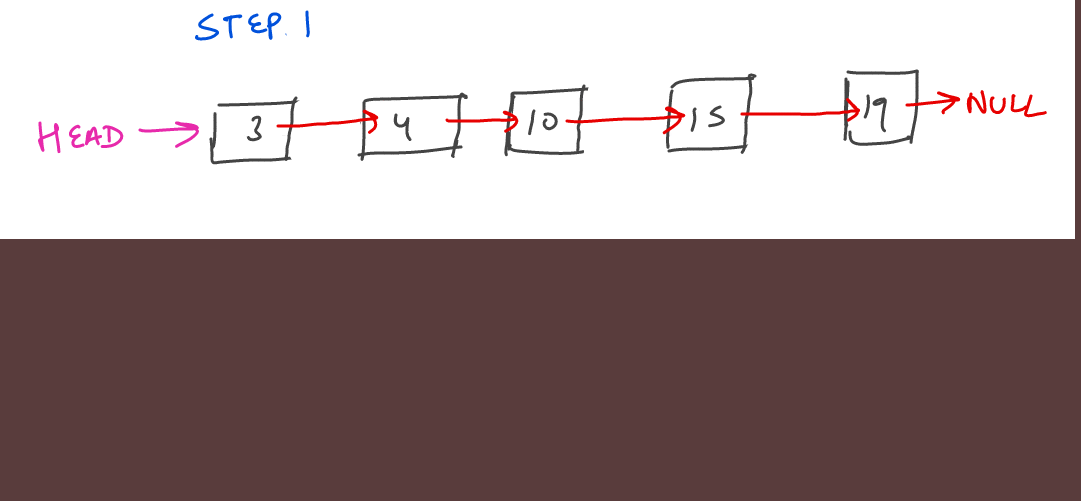# Rotating a singly linked list

Given a singly linked list and a number k. (k can be larger than n) Rotate the linked list k times.

#### Example Test Cases

##### Sample Test Case 1

Input Linked List: ` 3 -> 4 -> 10 -> 2 -> 15 ->19 `
K: ` 1 `
Expected Output: ` 19 -> 3 -> 4 -> 10 -> 2 -> 15 `
Explanation: This is because after 1 rotation ` 19 ` will come to the forward

##### Sample Test Case 2

Input Linked List: ` 3 -> 4 -> 10 -> 2 -> 15 ->19 `
K: ` 2 `
Expected Output: ` 15 -> 19 -> 3 -> 4 -> 10 -> 2 ->15`
Explanation: This is because:

1. After 1st rotation Linked List will become: ` 19 -> 3 -> 4 -> 10 -> 2 -> 15 `
2. After 2nd rotation Linked List will become: ` 15 -> 19 -> 3 -> 4 -> 10 -> 2 `

#### Solution

If we observe carefully, we will notice two things :

• Rotating a list n times becomes brings it back to it’s original form. Therefore, even if k is larger than n, we can just rotate the list k%n times and it will still print the same output. for example if ` n = 5, k = 1 ` and ` n = 5 and k = 6 ` will end up rotating the list in the same way. So it is better to rotate the list ` 6%5 = 1 ` times instead of 6.
• Rotating the list ` k ` times can be done by just rewiring the original linked list (changing the pointers for few of the nodes), instead of actually deleting elements from the end and inserting at the front. See the below diagram for better understanding
##### Rewiring

Rewiring the list can be done in the following steps

• Step 1: First calculate the length of the linked list. Let it be ` n `. Also do ` k = k%n `. To make sure that ` k < n ` going forward. In this case, ``` n = 5, k = 2 ```.
• Step 2: Find the last node at index ` (n - 1) ` and the node which will become the last node after rotation. Let us call that node ` before `. It will be located at index ` n - 1 - k `
• Step 3: Make the last node’s ` next pointer ` point towards the first node
• Step 4: Change the ` head ` to point to the node pointed to by ` before `. This way we are able to change the starting node of the linked list
• Step 5: Make the ` before ` node point to NULL, since the ` before ` node will become last in the final output.

See the below images for the visualization of the whole process:#### Implementation

```#include <iostream>
using namespace std;

typedef struct node {
int val;
node* next;
} node;

int findSizeOfList(node* head) {
node* curr = head;
int size = 0;
while (curr != NULL) {
size++;
curr = curr->next;
}
return size;
}

// Gives the nth node of the linked list.
node* findNode(node* head, int n) {
node* curr = head;
for(int i = 0; i < n; i++) {
curr = curr->next;
}
return curr;
}

// Helper method
void printLinkedList(node* head) {
node* curr = head;
while (curr != NULL) {
cout << curr->val << " ";
curr = curr->next;
}
cout << "\n";
}

// Helper method
void insertAtTheEnd(node** head, int val) {
node* newNode = new node;
newNode->val = val;
newNode->next = NULL;
if (*head == NULL) {
*head = newNode;
}
else {
node* curr = *head;
while (curr -> next != NULL) {
curr = curr->next;
}
curr->next = newNode;
}
}

void rotateRight(node** head, int k) {
int n = findSizeOfList(*head);
// After n rotations we get the same list, so rotating n + 1 times is same as rotating 1 time.
int newK = k%n;
if (newK > 0) {
// Last element after rotation
node* before = findNode(*head, n - 1 - newK);
// Current last node
node* lastNode = findNode(*head, n - 1);

// Step 3
lastNode->next = *head;
// Step 4
*head = before->next;
// Step 5
before->next = NULL;
}
}

int main() {
// Constructing the list
node* head = NULL;
insertAtTheEnd(&head, 3);
insertAtTheEnd(&head, 4);
insertAtTheEnd(&head, 10);
insertAtTheEnd(&head, 15);
insertAtTheEnd(&head, 19);

cout << "Printing before rotation\n";
printLinkedList(head);

rotateRight(&head, 2);

cout << "Printing after rotation\n";
printLinkedList(head);
return 0;
}```
Scroll to Top

### Full Stack Integrated Bootcamp Free Trial

• Please enter a number from 7000000000 to 9999999999.